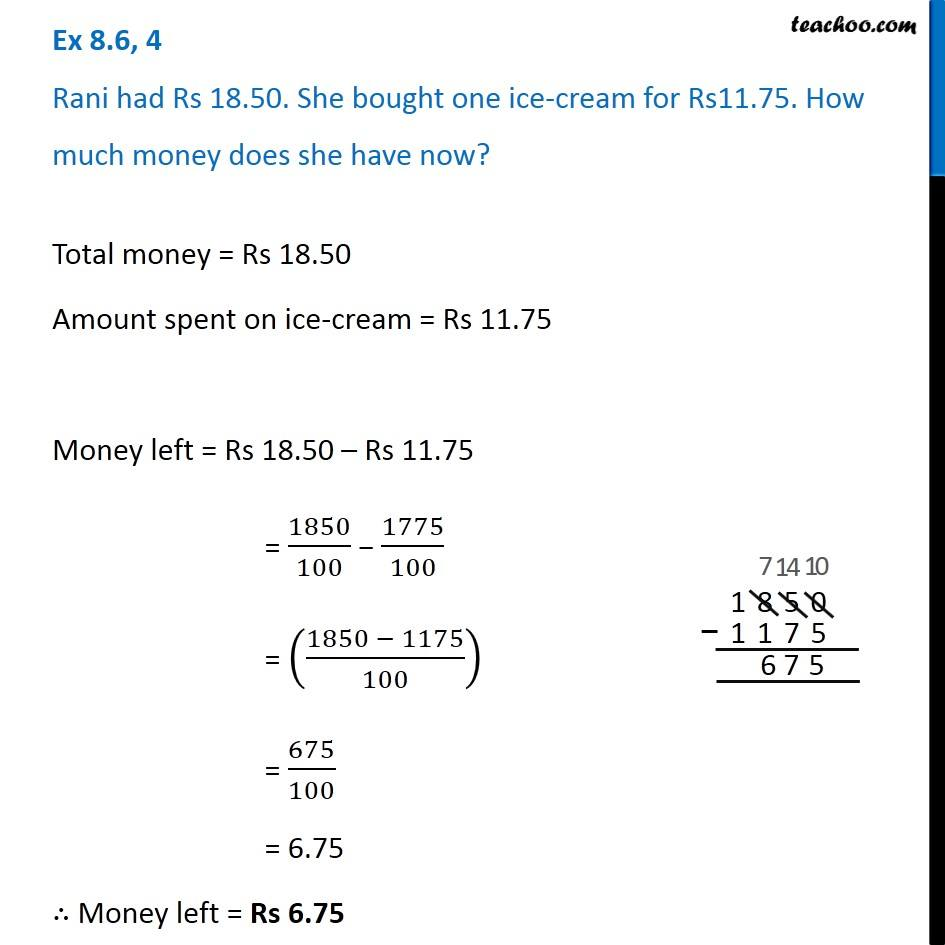1. Chapter 8 Class 6 Decimals
2. Serial order wise
3. Ex 8.6

Transcript

Ex 8.6, 4 Rani had Rs 18.50. She bought one ice-cream for Rs11.75. How much money does she have now?Total money = Rs 18.50 Amount spent on ice-cream = Rs 11.75 Money left = Rs 18.50 – Rs 11.75 = 1850/100 − 1775/100 = ((1850 − 1175)/100) = 675/100 = 6.75 ∴ Money left = Rs 6.75